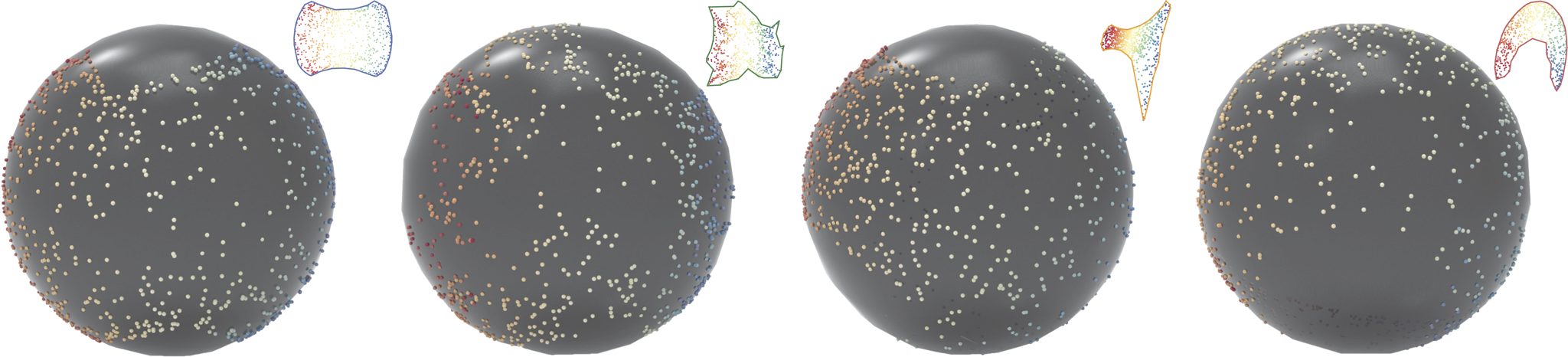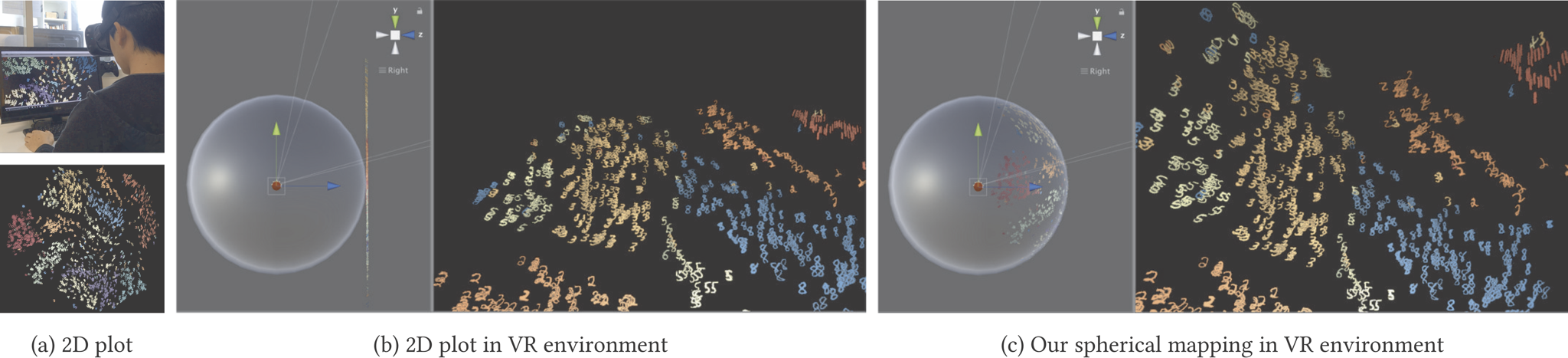## Abstract

In this paper, we present a technique that maps 2D plots to a spherical surface. We project 2D plots as large as possible while minimizing distortions. To accomplish this projection, we employ elliptical grid mapping, which maps points on a cube to those on a sphere. To determine the largest projection from points on the 2D plot to the cube, we find the largest projection inside an unfolded cube pattern. The unfolded cube is then folded to construct a cube, and the projected 2D plot is mapped to a spherical surface. We consider all unfolding patterns of a cube and determine the optimal pattern for the projection. We apply the proposed mapping to high-dimensional data visualizations. Since the technique maps a plot to a spherical surface surrounding the user, and this covers a wider range of the users field of view, our mapping provides an immersive experience to users.

## ResultsVisualization of the MNIST dataset in a VR environment. (a) Visualization of 2500 digit images by t-SNE. (b) Viewing of (a) in a VR environment. (c) Viewing of (a) mapped on a spherical surface by the proposed method.

## Acknowledgements

We thank all reviewers for their helpful comments. This work was supported by JSPS KAKENHI Grant number 19K24338.

## Bibtex

```@article{KITA2020101067,
title = "Mapping two-dimensional plots to a spherical surface using elliptical grid mapping",
journal = "Graphical Models",
volume = "109",
pages = "101067",
year = "2020",
issn = "1524-0703",
doi = "https://doi.org/10.1016/j.gmod.2020.101067",
url = "http://www.sciencedirect.com/science/article/pii/S1524070320300126",
author = "Naoki Kita and Grégoire Cliquet and Kazunori Miyata",
keywords = "Mapping technique, Layout optimization, Data visualization, Virtual reality",
abstract = "In this paper, we present a technique that maps 2D plots to a spherical surface. We project 2D plots as large as possible while minimizing distortions. To accomplish this projection, we employ elliptical grid mapping, which maps points on a cube to those on a sphere. To determine the largest projection from points on the 2D plot to the cube, we find the largest projection inside an unfolded cube pattern. The unfolded cube is then folded to construct a cube, and the projected 2D plot is mapped to a spherical surface. We consider all unfolding patterns of a cube and determine the optimal pattern for the projection. We apply the proposed mapping to high-dimensional data visualizations. Since the technique maps a plot to a spherical surface surrounding the user, and this covers a wider range of the users field of view, our mapping provides an immersive experience to users."
}
```